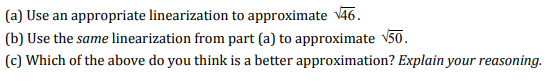# (a) Use an appropriate linearization to approximate 46(b) Use the same linearization from part (a) to approximate N50(c) Which of the above do you think is a better approximation? Explain your reasoning.

Questionhelp_outlineImage Transcriptionclose(a) Use an appropriate linearization to approximate 46 (b) Use the same linearization from part (a) to approximate N50 (c) Which of the above do you think is a better approximation? Explain your reasoning. fullscreen
check_circle

Step 1

For a given function f(x), the equation of tangent at a point x = a and y = f(a) is given by:

y - f(a) = f'(a).(x - a)

Hence, y = f(a) + f'(a).(x - a)

Also, since y = f(x), we can rewrite the above equation as:

f(x) = f(a) + f'(a).(x - a)

This is the linearization function for f(x), which is linear in nature with respect to x.

Step 2

Part (a)

We have to find (46)1/2

So, the corresponding function will be f(x) =x1/2

We now need to choose "a". Let's choose "a" in close vicinity of 46 for which square root is easy to find.

Hence, let's choose a = 49

f(a) = f(49) = 491/2 = 7

f'(x) = 0.5x-1/2

f'(a) = 0.5 x 49-1/2 = 0.5 / 7 = 0.071428571 = 0.0714

Hence, f(x) = f(a) + f'(a).(x - a)

f(x) = x1/2 = 7 + 0.0714 (x - 49)

Now set x = 46.

Hence,

461/2 = 7 + 0.0714 x (46 – 49) = 6.785714286 = 6.7857

Step 3

Part (b)

Now set x = 50.

Hence,

461/2 = 7 + 0.0714 x...

### Want to see the full answer?

See Solution

#### Want to see this answer and more?

Solutions are written by subject experts who are available 24/7. Questions are typically answered within 1 hour.*

See Solution
*Response times may vary by subject and question.
Tagged in

### Derivative